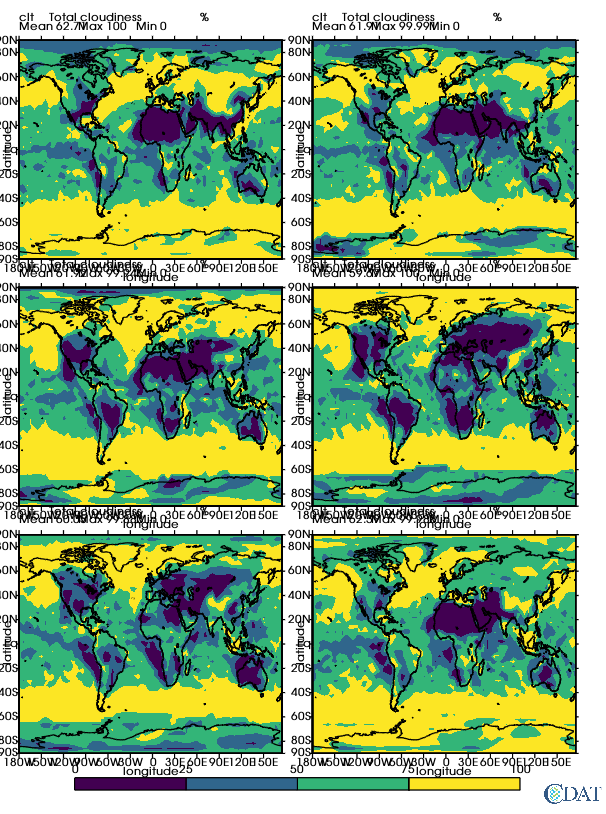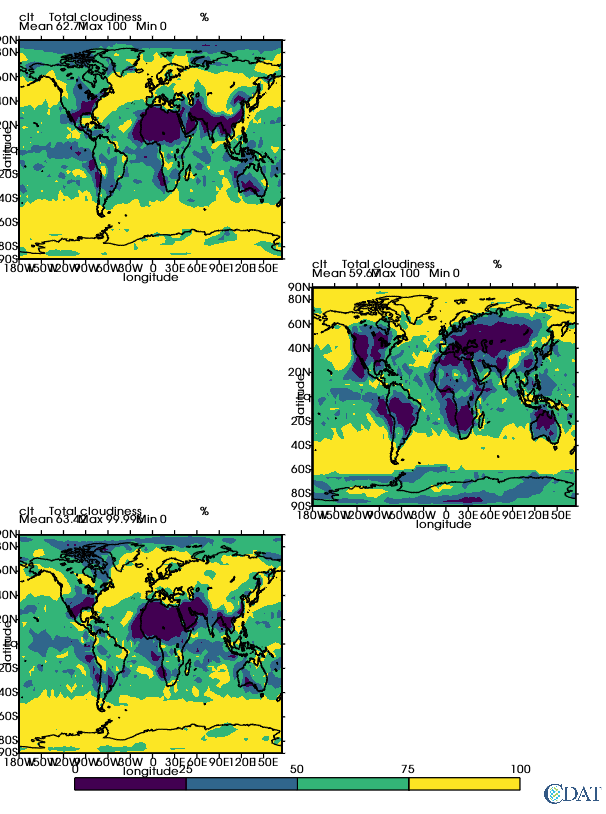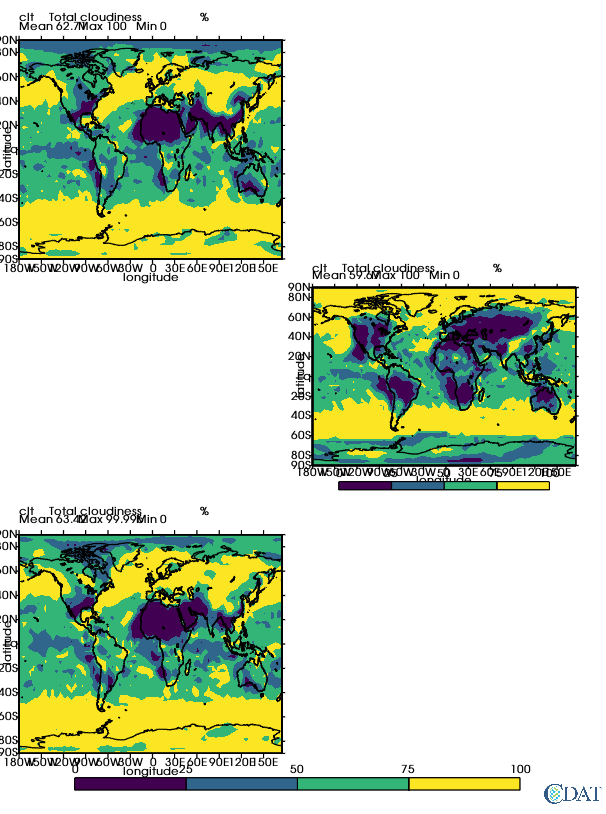# Ez Templates¶

This tutorial shows how to easily create templates for making images with multiple plots using CDAT's VCS tool.

To download this Jupyter Notebook, right click on the link and choose "Download Linked File As..." or "Save Link as...". Remember where you saved the downloaded file, which should have an .ipynb extension. (You'll need to launch the Jupyter notebook or JupyterLab instance from the location where you store the notebook file.)

# Introduction¶

VCS's template objects allow users to have multiple plots on a single page or a single canvas. Tweaking the templates can be tedious, however. This is where the EzTemplate comes handy since it helps create a VCS template object for the most common plot configurations.

# Setting Up the Notebook¶

In :
from __future__ import division, print_function
from IPython.display import Image
import vcs
import cdms2

canvas = vcs.init(bg=True)
f = cdms2.open(vcs.sample_data+"/clt.nc")
clt = f("clt")
gm = vcs.createisofill()
levels = list(range(0,110,25))
gm.levels = levels
gm.fillareacolors = vcs.getcolors(levels)

box = vcs.createline()
box.x = [0.001, .999, .999, .001, .001]
box.y = [0.001, .001, .999, .999, .001]

def plot_all(M):
canvas.clear()
for i in range(M.rows * M.columns):
template = M.get(column = i % M.columns, row = i // M.columns) # This is the VCS template object you could further edit
display = canvas.plot(clt[i*2], template, gm)
return canvas.plot(box)

Downloading: 'BlueMarble.ppm' from 'https://cdat.llnl.gov/cdat/sample_data/' in: /Users/davis278/miniconda3/envs/cdat81-mesa/share/cdat/sample_data/BlueMarble.ppm


# Creating Templates for 2x3 Plots¶

Let's say we want to have 6 plots, divided into 3 rows of 2 plots per row. Notice the canvas automatically switches to a portrait orientation.

In :
Multiple = EzTemplate.Multi(rows=3, columns=2, x=canvas)
for i in range(6):
template = Multiple.get()  # This is the VCS template object you could further edit
display = canvas.plot(clt[i*2], template, gm)
display  # To render final image in the notebook

Out:# Accessing a Specific Template¶

Rather than accessing templates in order, you can retrieve a specific object directly.

In :
canvas.clear()
template = Multiple.get(row=0, column=0)  # This is the VCS template object you could further edit
canvas.plot(clt, template, gm)
template = Multiple.get(row=1, column=1)  # This is the VCS template object you could further edit
canvas.plot(clt, template, gm)
template = Multiple.get(row=2, column=0)  # This is the VCS template object you could further edit
canvas.plot(clt, template, gm)

Out:# Subplot Legends¶

You probably noticed that only one legend is plotted. Sometimes you might want a separate legend for a specific subplot. This can be achieved when you get the template.

In :
canvas.clear()
canvas.clear()
template = Multiple.get(row=0, column=0)  # This is the VCS template object you could further edit
canvas.plot(clt, template, gm)
template = Multiple.get(row=1, column=1, legend="local")  # This is the VCS template object you could further edit
canvas.plot(clt, template, gm)
template = Multiple.get(row=2, column=0)  # This is the VCS template object you could further edit
canvas.plot(clt, template, gm)

Out:# Controlling Subplot Aspects¶

Many general aspects of the plot can be controlled via the Multi object. A list of controllable aspects and their current values can be accessed via the list() function.

In :
Multiple.list()

----------Template (P) member (attribute) listings ----------
rows = 3
columns = 2
template = default
member = margins
top = 0.05
bottom = 0.075
right = 0.05
left = 0.033
member = spacing
horizontal = 0.05
vertical = 0.035
member = legend
direction = horizontal
stretch = 0.8
thickness = 0.2
fat = 0.05


## Base Template¶

By default, each individual template is generated based on the default VCS template. You can pass your template the base template you would like to be used by default.

In :
base_template = vcs.createtemplate()
base_template.blank(["title","crtime","crdate",
"source","dataname", "units",
"tname", "tvalue", "zvalue",
"min", "max",
"xname", "xlabel1"])
canvas.clear()
canvas.plot(clt,base_template, gm)

Out: